# Cutting Slip Velocity Calculation Method 1

Cutting slip velocity is velocity of cutting that naturally falls down due to its density. In order to effectively clean the hole, effect of mud flow upward direction and mud properties must be greater than cutting slip velocity (settling tendency of cuttings). Otherwise, cutting will fall down and create cutting bed.

This calculation will show annular velocity, cutting slip velocity and net velocity so you can use as a reference for you hole cleaning indication.

There are 2 calculation methods and I will show the first method via this topic.

1. Determine annular velocity with following equation: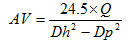Where;

AV is annular velocity in ft/min.

Q is flow rate in gpm (gallon per minute).

Dh is diameter of hole in inch.

Dp is diameter of drill pipe in inch.

2. Determine cutting slip velocity with following equation: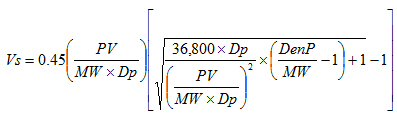Where;

Vs is cutting slip velocity in ft/min.

PV is plastic viscosity in centi-poise.

MW is mud weight in ppg.

Dp is diameter of cutting in inch.

DenP is cutting density in ppg.

3. Determine net rise velocity with following equation:

Net rise velocity = AV – Vs

Where;

AV is annular velocity in ft/min.

Vs Cutting Velocity in ft/min.

This figure indicates that cuttings are being lifted by mud or are still falling down.

If net rise velocity is positive, it means that you have good flow rate which can carry cutting in the wellbore.

On the other hand, If net rise velocity is negative, your current flow rate is NOT engough to carry cuttings.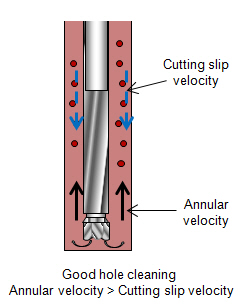Example: Please use the following information to determine annular velocity, cutting slip velocity, net rise velocity, and tell us if the flow rate is good for hole cleaning.

Information

Flow rate = 300 gpm

Hole Diameter = 6.3 inch

Drillpipe OD = 4 inch

PV = 15 cps

MW = 10 ppg

Diameter of cutting = 0.20 inch

Density of cutting = 20.0 ppg

1. Determine annular velocity: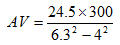AV = 310.3 ft/min

2. Determine cutting slip velocity: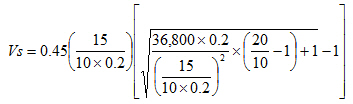Vs = 35.4 ft/min

3. Determine net rise velocity with following equation:

Net rise velocity = AV – Vs

Net rise velocity = 310.3 – 35.4 = 274.9 ft/min

Conclusion: This flow rate is GOOD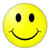for hole cleaning practice because annular velocity is more than cutting slip velocity.

Share the joyWorking in the oil field and loving to share knowledge.

### 5 Responses to Cutting Slip Velocity Calculation Method 1

1.Johnson Okoronkwo says:

I am a second Mud Engineer,i like your site . Please keep it on . Thank you .

2.dado awad says:

HI, i am asking about slip velocity , in order to perform good hole cleaning should i calculate the slip velocity or only i should calculate the annulus velocity which is enough to let me perform good hole cleaning & cutting lifting out of hole.

•DrillingFormulas.Com says:

If you can, I recommend you to determine the slip velocity.

3.ed hidi says:

It is important to calculate cut slip velocity, because it is variable of depth, bit type, instrument rotation, formation texture etc.

This site uses Akismet to reduce spam. Learn how your comment data is processed.